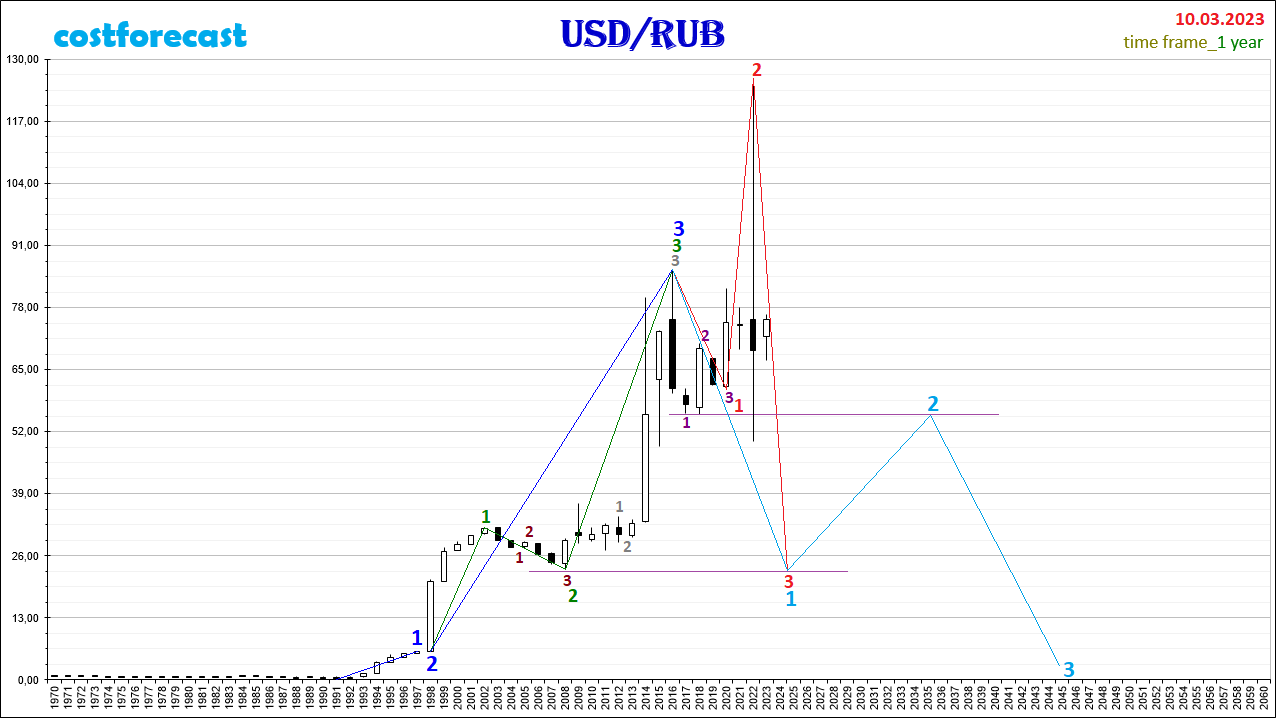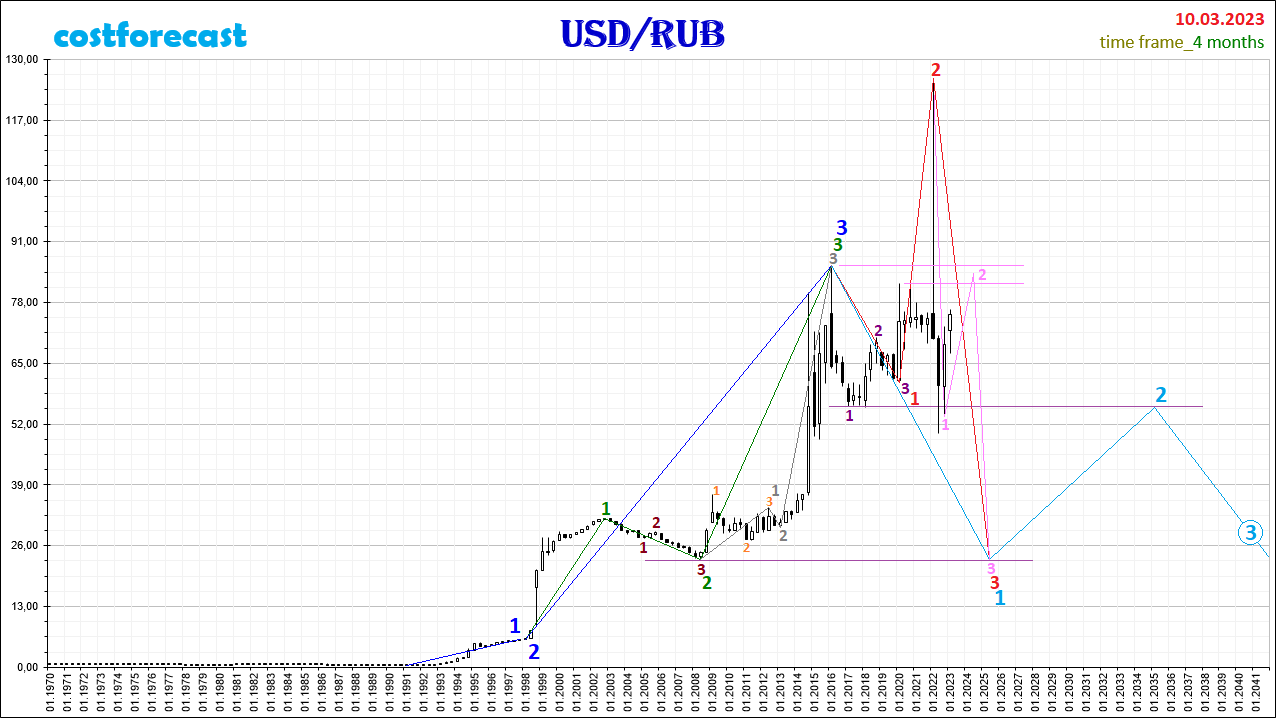# Ruble, forecast 03/10/2023. – Analytics & Forecasts – 17 March 2023

The fractal structure of the chart with a time period of 1 year.

Here you can see the forecast of the dynamics of the exchange rates of the ruble a year ago.From 03/10/2023, it can be assumed that in the fractal structure of the chart of dynamics of quotes of currency pairs USD/RUB during the time interval (2020; 2022) at the point with coordinates (03/10 /2022; 126.00) , the 2nd segment of the fractal, which is marked in red, and that the further drop between quotes occurs as part of the formation of the 3rd segment of this red fractal.

The fractal marked in red is the F14 fractal, i.e. View 1 and Type 4 (see Alphabet of Niro attractions). The first segment of the red fractal is fractal F33 (3rd view, 3rd type), the segments of which are indicated on the chart with purple numbers and has a time interval (2016, 2020). In this case, the current dynamics of USD/RUB quotes will be down as part of the formation of the third segment of the red fractal in the time interval (2022, 2025).

The assumption that a fractal is formed in the fractal structure of the USD/RUB chart, which is marked in red on the chart, is based on the fact that the fractal F11 (view 1, type 1) was formed in the interval of time (1991.2016). ), which is marked in blue.

The 1st segment of the fractal, marked in blue, was formed in the time interval (1991,1997). The time interval of the 2nd segment of the blue fractal is the interval (1997, 1998). The third segment of the blue fractal was formed in the time interval (1998, 2016).

The blue fractal ended in 2016 with its third segment formed in the time interval (1998, 2016), which is indicated on the chart by the green fractal F11 (view 1, type 1).

The second segment of the green fractal is fractal F21 (view 2, type 1), whose segments are indicated on the graph with dark brown numbers within the time interval (2002, 2008). The third segment of the green fractal was formed in the time interval (2008, 2016) as fractal F11 (view 1, type 1), whose segments are marked with gray numbers.

Taking into account the above, the current dynamics of the quotations of the currency pair USD/RUB will be downward towards the mark of 23 rubles per dollar in 2025 as part of the completion of the formation of the red fractal, which is the first segment. of the higher order fractal, which is indicated in the graph in blue.

After 2025, the dynamics of the quotations of the currency pair USD/RUB will be upward until 2035 as part of the formation of the second segment of the blue fractal in the direction of 55 rubles per dollar.Analyzing the fractal structure in the graph of the dynamics of the ruble quotations, constructed with a time period of 4 months, we can assume that the downward dynamics will probably occur within the formation of a fractal, which is indicated in rose Given this hypothesis, at the point with coordinates (30/09/2022; 53.64), the 1st segment of the pink fractal was completed and its 2nd segment began to form, suggesting an increase in comets to the mark located in the range. (81.98; 85, 99), after which the downward dynamics will continue as part of the formation of the third segment of the pink fractal in the direction of 23.00.